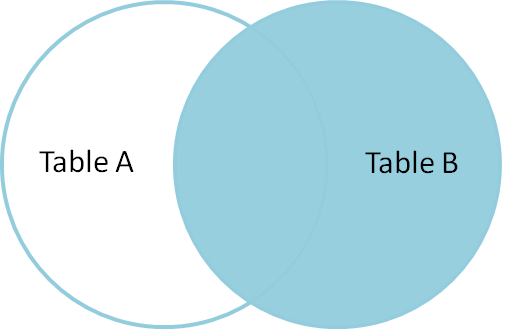Hello Guys! This is my first blog post of this month and in this post I am going to explain about SQL Joins which is considered to be a very integral topic of SQL. The examples shown in this post have been taken from www.tutorialspoint.com.

SQL join is a clause which is used to combine records/rows from two or more tables in a database. There are various types of joins: INNER, LEFT OUTER, RIGHT OUTER, FULL OUTER and CROSS. A table can also JOIN to itself which is known as a self-join.

Consider the following two tables, CUSTOMERS table is as follows:

INNER JOIN is a very common type of join that is frequently used in SQL. Inner join matches rows from two tables based on the values in common columns from each table. For example, retrieving all rows where the student roll number is the same in both the students and courses tables.

An inner join gives the intersection of the two tables, i.e. the two rows they have in common.LEFT JOIN fetches all rows from the left table, even if there is no matching row in the right table but with NULL value in each column from right table.

So the left join returns all the values from the left table including matched values from the right table or NULL in case there is no match.

Below is the Venn Diagram for SQL left join:-RIGHT JOIN fetches all rows from the right table, even if there is no matching row in the left table but with NULL value in each column from left table.

So the right join returns all the values from the right table including matched values from the left table or NULL in case there is no match.

Below is the Venn Diagram for SQL right join:-That was all about SQL Joins.
I hope you liked it. 🙂